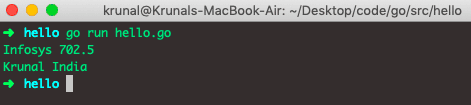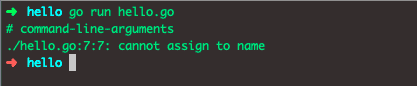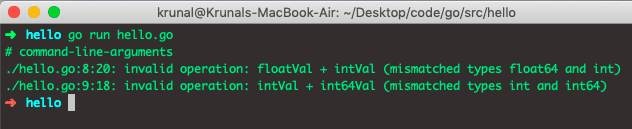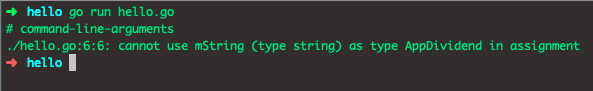# Golang Constant: The Complete Guide

Go supports the constants of character, string, boolean, and numeric values. Constants are created at compile time, even when defined as locals in functions, and can only numeric characters (runes), strings, or booleans.

Because of Golang’s compile-time restriction, the expressions that define them must be constant expressions evaluated by the compiler.

## Golang constant

The const in Golang declares a constant value. Golang uses a term constant to represent fixed (unchanging) values such as 3.14, 21, “Hello,” etc. Constant expressions perform arithmetic with arbitrary precision.

The numeric constant has no type until it’s given, such as by an explicit cast. A number can be given the type by using it in a context that requires one, such as the variable assignment or a function call.

Literals are constants. All the literals in Go, be it integer literals like 21, 46, or floating-point literals like 3.14, 19.21, or boolean literals like true, false, or string literals like “Krunal,”Ankit” are constants.

## How to declare a constant in Golang

To declare a constant in Golang, use the keyword const and give it a name.

```const blog = "AppDividend"
const flag = true```

You can also specify the type in the declaration, like the following code.

```const x int = 1921
const y string = "Krunal"```

We can also define multiple constants. See the following code.

```// hello.go

package main

import "fmt"

func main() {
const name, country = "Krunal", "India"

const (
stockName  string  = "Infosys"
stockPrice float64 = 702.50
)
fmt.Println(stockName, stockPrice)
fmt.Println(name, country)
}
```

See the below output.Now, let’s try to modify the value of the constants and see the result.

```// hello.go

package main

import "fmt"

func main() {
const name, country = "Krunal", "India"
name = "Ankit"
fmt.Println(name, country)
}
```

See the below output.So, we have got the error like Cannot assign the constant.

## Typed and untyped constants in Golang

Go is the statically typed programming language which means that a type of every variable is known or inferred by the compiler at the compile time. But it goes beyond that step with its type system and doesn’t even allow us to perform the operations that mix numeric types.

For example, We cannot add the float64 variable to an int or an int64 variable to an int. See the following code.

```// hello.go

package main

func main() {
var floatVal float64 = 3.14
var intVal int = 19
var int64Val int64 = 21

var o1 = floatVal + intVal
var o2 = intVal + int64Val
}
```

See the below output.So, we have got the error like invalid operation. Golang doesn’t provide an implicit type conversion and requires you to do the explicit type casting whenever we mix the variables of multiple types in the operation.

## Untyped Constants in Golang

Any constant in Go, named or unnamed, is untyped unless given a type explicitly. See the following examples.

```21       // untyped integer constant
1.9     // untyped floating-point constant
false    // untyped boolean constant
"Cloud"```

See the untyped constants even after you give the name.

```const x = 19
const y = 2.1
const z = false
const w = "Zing"```

Let’s now see the example of an untyped string constant.

In Go, you can create the type alias using the type keyword like the following.

`type AppDividend string`

Given the strongly typed nature of the Golang, you can’t assign the string variable to an AppDividend variable.

```// hello.go

package main

func main() {
type AppDividend string
var mString string = "Hello"
var mStringA AppDividend = mString
}
```

See the below output.Untyped constants are a fantastic design decision taken by Go creators. Although Go has a robust type system, You can use the untyped constants to escape from the Go’s type system and have more flexibility when working with mixed data types in any expression.

## Typed Constants in Golang

In Go, Constants are typed when you specify a type in the declaration like the following syntax.

`const typedInt int = 21`

Just like the variables, all the rules of Go’s type system apply to the typed constant. For example, you cannot assign the typed integer constant to a float variable. See the following code.

`var myFloat64 float64 = typedInt  // Compiler Error`

With the typed constants, you lose all the flexibility that comes with the untyped constants, like assigning them to any variable of the compatible type or mixing them in mathematical operations. So you should declare the type for a constant only if necessary. Otherwise, declare constants without a type.

That’s it for this tutorial.

Categories Go

This site uses Akismet to reduce spam. Learn how your comment data is processed.